# Simplified molecular input line entry specification facts for kids

Kids Encyclopedia Facts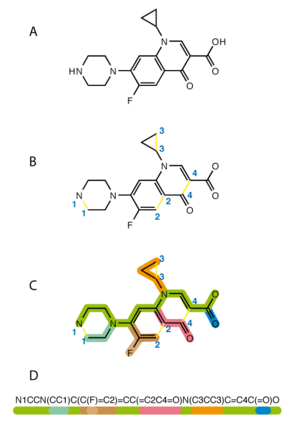Steps in writing a SMILES code: Break cycles, then write as branches off a main backbone.

The simplified molecular input line entry specification or SMILES is a line notation for the chemical structure of molecules. It uses a short series ASCII characters to represent structures. Most molecule editor computer programs can draw a two-dimensional diagram or a three-dimensional model of a molecule based on its SMILES code.

Arthur Weininger and David Weininger wrote the original SMILES specification in the late 1980s. It has since been modified and extended by others, including by Daylight Chemical Information Systems Inc. In 2007, the Blue Obelisk open-source chemistry community developed an open standard called "OpenSMILES".

In July 2006, the IUPAC introduced the InChI as a standard line notation for representing chemical structures. People think that it is easier for humans to understand SMILES than InChI. Some chemists argue that SMILES is better than InChI because many different software programs support it. SMILES also has theoretical (for example, graph theory) backing.

## Conversion

SMILES can be converted back to 2-dimensional representations using Structure Diagram Generation algorithms. This conversion can sometimes be ambiguous. Because SMILES just says which atoms are connected without any information about the angles between chemical bonds, computer programs use energy minimization to convert SMILES to 3-dimensional representations. There are many downloadable and web-based conversion utilities.

## Examples

Here are some examples of the SMILES for molecules:

Molecule Structure SMILES Formula
Dinitrogen N≡N N#N
Methyl isocyanate (MIC) CH3–N=C=O CN=C=O
Copper(II) sulfate Cu2+ SO42- [Cu+2].[O-]S(=O)(=O)[O-]
Œnanthotoxin (C17H22O2)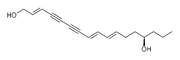CCC[C@@H](O)CC\C=C\C=C\C#CC#C\C=C\CO
Pyrethrin II (C22H28O5)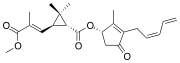COC(=O)C(\C)=C\C1C(C)(C)[C@H]1C(=O)O[C@@H]2C(C)=C(C(=O)C2)CC=CC=C
Aflatoxin B1 (C17H12O6)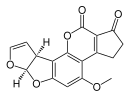O1C=C[C@H]([C@H]1O2)c3c2cc(OC)c4c3OC(=O)C5=C4CCC(=O)5
Glucose (glucopyranose) (C6H12O6)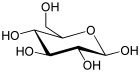OC[C@@H](O1)[C@@H](O)[C@H](O)[C@@H](O)[C@@H](O)1
Bergenin (cuscutin) (a resin) (C14H16O9)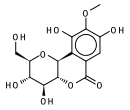OC[C@@H](O1)[C@@H](O)[C@H](O)[C@@H]2[C@@H]1c3c(O)c(OC)c(O)cc3C(=O)O2
A pheromone of the Californian scale insect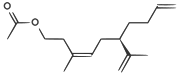CC(=O)OCCC(/C)=C\C[C@H](C(C)=C)CCC=C
2S,5R-Chalcogran: a pheromone of the bark beetle Pityogenes chalcographusCC[C@H](O1)CC[C@@]12CCCO2
Vanillin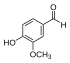O=Cc1ccc(O)c(OC)c1
Melatonin (C13H16N2O2)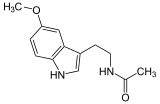CC(=O)NCCC1=CNc2c1cc(OC)cc2
Flavopereirin (C17H15N2)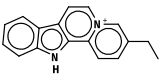CCc(c1)ccc2[n+]1ccc3c2Nc4c3cccc4
Nicotine (C10H14N2)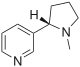CN1CCC[C@H]1c2cccnc2
Alpha-thujone (C10H16O)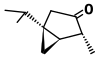CC(C)[C@@]12C[C@@H]1[C@@H](C)C(=O)C2
Thiamin (C12H17N4OS+)
(vitamine B1)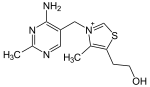OCCc1c(C)[n+](=cs1)Cc2cnc(C)nc(N)2
• Weininger, David (1988). "SMILES, a chemical language and information system. 1. Introduction to methodology and encoding rules". Journal of Chemical Information and Modeling 28: 31. doi:10.1021/ci00057a005.
• Weininger, David; Weininger, Arthur; Weininger, Joseph L. (1989). "SMILES. 2. Algorithm for generation of unique SMILES notation". Journal of Chemical Information and Modeling 29 (2): 97. doi:10.1021/ci00062a008.
• Helson, H.E. (1999). "Structure Diagram Generation". In Lipkowitz, K. B. and Boyd, D. B.. Rev. Comput. Chem.. 13. New York: Wiley-VCH. pp. 313–398. doi:10.1002/9780470125908.ch6.

### SMILES related software utilitiesSimplified molecular input line entry specification Facts for Kids. Kiddle Encyclopedia.